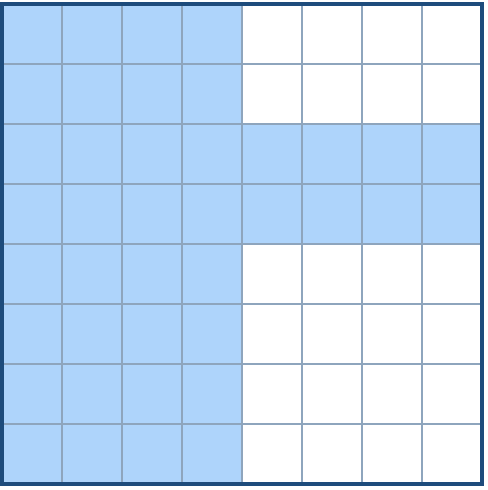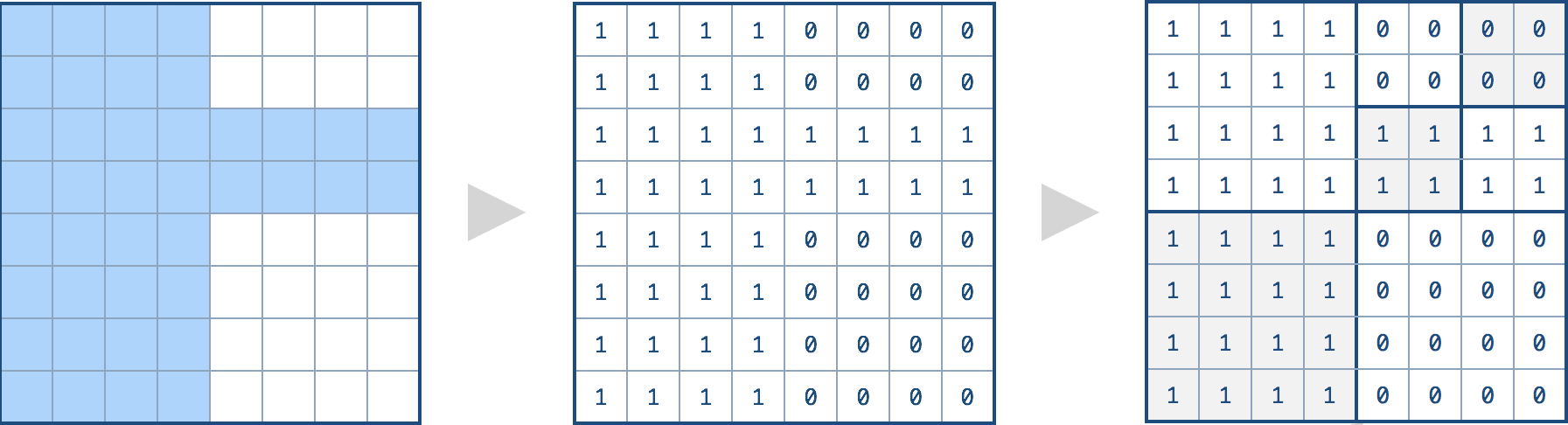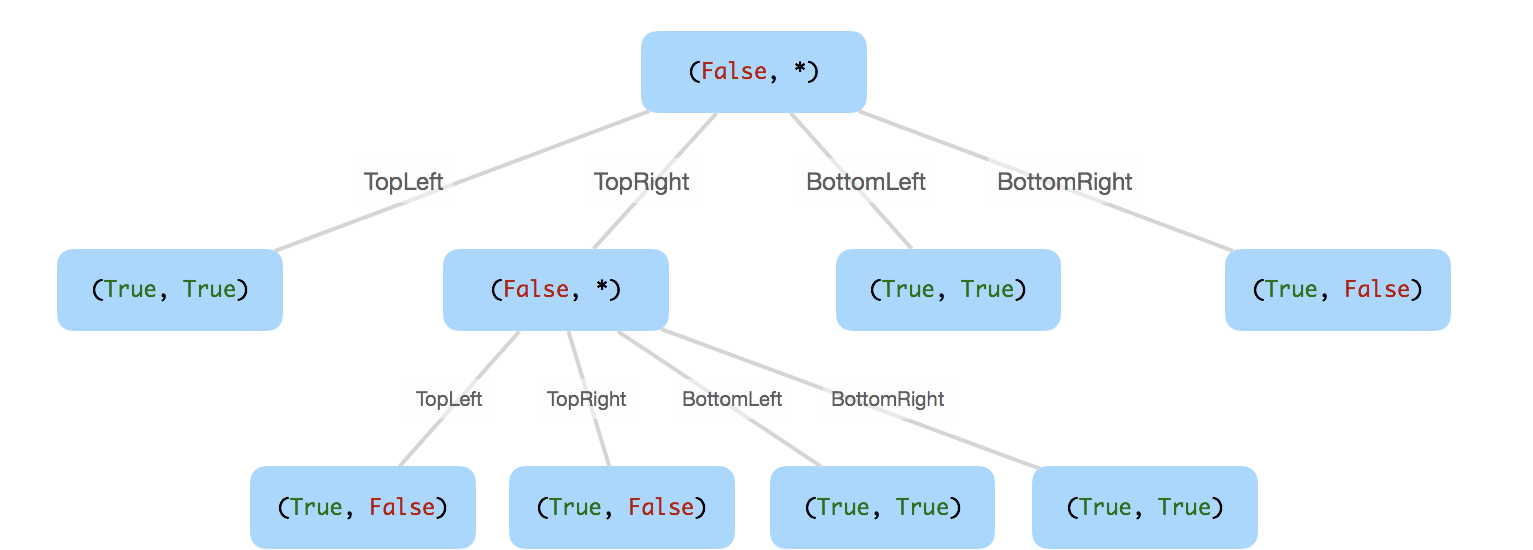# leetcode 427. Construct Quad Tree

2018/07/23 22:57

We want to use quad trees to store an `N x N` boolean grid. Each cell in the grid can only be true or false. The root node represents the whole grid. For each node, it will be subdivided into four children nodes until the values in the region it represents are all the same.

Each node has another two boolean attributes : `isLeaf` and `val`. `isLeaf` is true if and only if the node is a leaf node. The `val` attribute for a leaf node contains the value of the region it represents.

Given the `8 x 8` grid below, we want to construct the corresponding quad tree:It can be divided according to the definition above:The corresponding quad tree should be as following, where each node is represented as a `(isLeaf, val)` pair.

For the non-leaf nodes, `val` can be arbitrary, so it is represented as `*`.Note:

1. `N` is less than `1000` and guaranteened to be a power of 2.
2. If you want to know more about the quad tree, you can refer to its wiki.
``````"""
# Definition for a QuadTree node.
class Node(object):
def __init__(self, val, isLeaf, topLeft, topRight, bottomLeft, bottomRight):
self.val = val
self.isLeaf = isLeaf
self.topLeft = topLeft
self.topRight = topRight
self.bottomLeft = bottomLeft
self.bottomRight = bottomRight
"""
class Solution(object):
def construct(self, grid):
"""
:type grid: List[List[int]]
:rtype: Node
"""
def construct2(g, i1, j1, n):
s = g[i1][j1]
is_same = True
for i in xrange(i1, i1+n):
for j in xrange(j1, j1+n):
if g[i][j] != s:
is_same = False
break
if is_same:
return Node(s, True, None, None, None, None)
else:
root = Node("*", False, None, None, None, None)
root.topLeft = construct2(g, i1, j1, n/2)
root.topRight = construct2(g, i1, j1+n/2, n/2)
root.bottomLeft = construct2(g, i1+n/2, j1, n/2)
root.bottomRight = construct2(g, i1+n/2, j1+n/2, n/2)
return root

return construct2(grid, 0, 0, len(grid))``````

``````class Solution:
def construct(self, grid):
def dfs(x, y, l):
if l == 1:
node = Node(grid[x][y] == 1, True, None, None, None, None)
else:
tLeft = dfs(x, y, l // 2)
tRight = dfs(x, y + l // 2, l // 2)
bLeft = dfs(x + l // 2, y, l// 2)
bRight = dfs(x + l // 2, y + l // 2, l // 2)
value = tLeft.val or tRight.val or bLeft.val or bRight.val
if tLeft.isLeaf and tRight.isLeaf and bLeft.isLeaf and bRight.isLeaf and tLeft.val == tRight.val == bLeft.val == bRight.val:
node = Node(value, True, None, None, None, None)
else:
node = Node(value, False, tLeft, tRight, bLeft, bRight)
return node
return grid and dfs(0, 0, len(grid)) or None``````

0
0 收藏

### 作者的其它热门文章0 评论
0 收藏
0